Deformable Convolutional Networks

1. 引言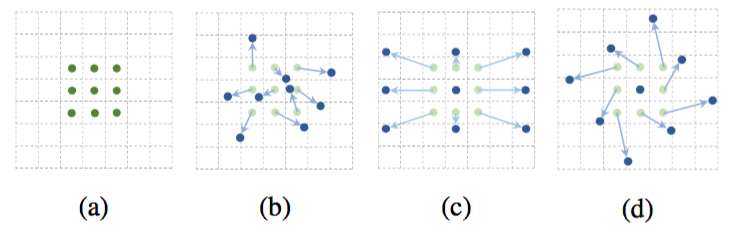2. 可变形卷积网络

CNN中的特征映射和卷积是3D的。可变形卷积和RoI池化模块都在2D空间域上运行。在整个通道维度上的操作保持不变。在不丧失普遍性的情况下，为了符号清晰，这些模块在2D中描述。扩展到3D很简单。

2.1. 可变形卷积

2D卷积包含两步：1）用规则的网格$\mathcal{R}$$\mathcal{R}$在输入特征映射$\mathbf{x}$$\mathbf{x}$上采样；2）对$\mathbf{w}$$\mathbf{w}$加权的采样值求和。网格$\mathcal{R}$$\mathcal{R}$定义了感受野的大小和扩张。例如，

$\mathcal{R}=\left\{\left(-1,-1\right),\left(-1,0\right),\dots ,\left(0,1\right),\left(1,1\right)\right\}$

$\begin{array}{}\text{(1)}& \mathbf{y}\left({\mathbf{p}}_{0}\right)=\sum _{{\mathbf{p}}_{n}\in \mathcal{R}}\mathbf{w}\left({\mathbf{p}}_{n}\right)\cdot \mathbf{x}\left({\mathbf{p}}_{0}+{\mathbf{p}}_{n}\right)\end{array}$

$\begin{array}{}\text{(2)}& \mathbf{y}\left({\mathbf{p}}_{0}\right)=\sum _{{\mathbf{p}}_{n}\in \mathcal{R}}\mathbf{w}\left({\mathbf{p}}_{n}\right)\cdot \mathbf{x}\left({\mathbf{p}}_{0}+{\mathbf{p}}_{n}+\mathrm{\Delta }{\mathbf{p}}_{n}\right).\end{array}$

$\begin{array}{}\text{(3)}& \mathbf{x}\left(\mathbf{p}\right)=\sum _{\mathbf{q}}G\left(\mathbf{q},\mathbf{p}\right)\cdot \mathbf{x}\left(\mathbf{q}\right),\end{array}$

$\begin{array}{}\text{(4)}& G\left(\mathbf{q},\mathbf{p}\right)=g\left({q}_{x},{p}_{x}\right)\cdot g\left({q}_{y},{p}_{y}\right),\end{array}$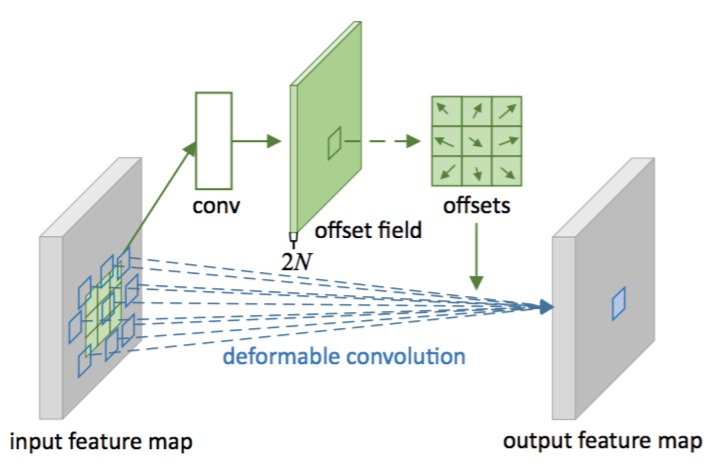2.2. 可变形RoI池化

RoI池化。给定输入特征映射$\mathbf{x}$$\mathbf{x}$、RoI的大小$w×h$$w\times h$和左上角${\mathbf{p}}_{0}$$\mathbf{p}_0$，RoI池化将ROI分到$k×k$$k\times k$$k$$k$是一个自由参数）个组块(bin)中，并输出$k×k$$k\times k$的特征映射$\mathbf{y}$$\mathbf{y}$。对于第$\left(i,j\right)$$(i,j)$个组块($0\le i,j$0\le i,j < k$)，我们有

$\begin{array}{}\text{(5)}& \mathbf{y}\left(i,j\right)=\sum _{\mathbf{p}\in bin\left(i,j\right)}\mathbf{x}\left({\mathbf{p}}_{0}+\mathbf{p}\right)/{n}_{ij},\end{array}$

$\begin{array}{}\text{(6)}& \mathbf{y}\left(i,j\right)=\sum _{\mathbf{p}\in bin\left(i,j\right)}\mathbf{x}\left({\mathbf{p}}_{0}+\mathbf{p}+\mathrm{\Delta }{\mathbf{p}}_{ij}\right)/{n}_{ij}.\end{array}$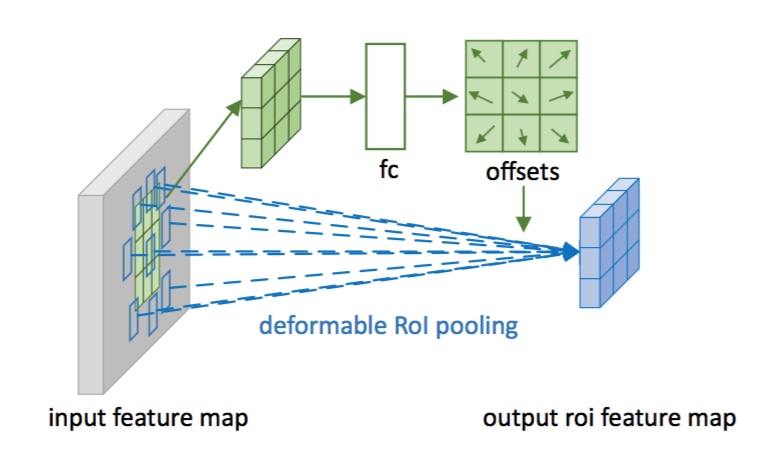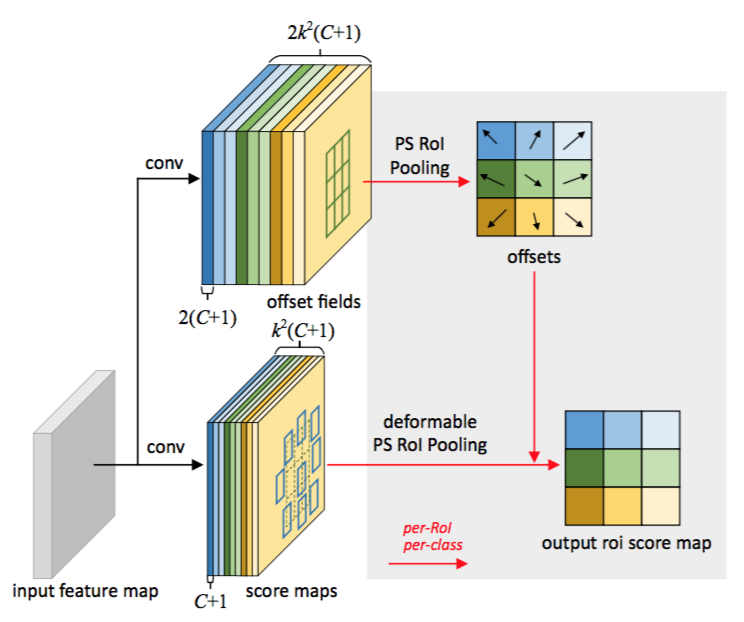2.3. 可变形卷积网络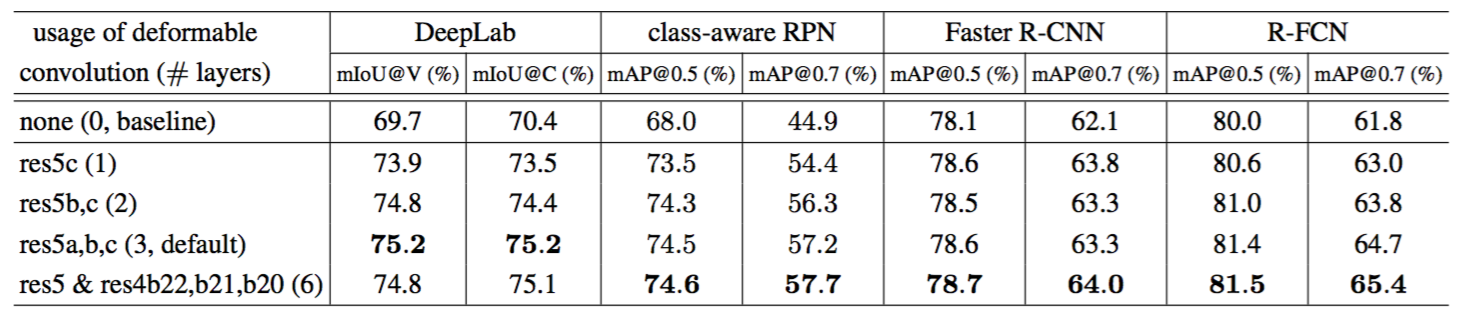DeepLab是最先进的语义分割方法。它在特征映射上添加1×1卷积层以生成表示每个像素分类分数的（C+1）个映射。然后随后的softmax层输出每个像素的概率。

Faster R-CNN是最先进的检测器。在我们的实现中，RPN分支被添加在conv4块的顶部，遵循。在以前的实践中[22,24]，在ResNet-101的conv4和conv5块之间插入了RoI池化层，每个RoI留下了10层。这个设计实现了很好的精确度，但是具有很高的per-RoI计算。相反，我们采用中的简化设计。RoI池化层在最后添加。在池化的RoI特征之上，添加了两个1024维的全连接层，接着是边界框回归和分类分支。虽然这样的简化（从10层conv5块到2个全连接层）会稍微降低精确度，但它仍然具有足够强的基准，在这项工作中不再关心。

R-FCN是另一种最先进的检测器。它的每个RoI计算成本可以忽略不计。我们遵循原来的实现。可选地，其RoI池化层可以改变为可变形的位置敏感的RoI池化

3. 理解可变形卷积网络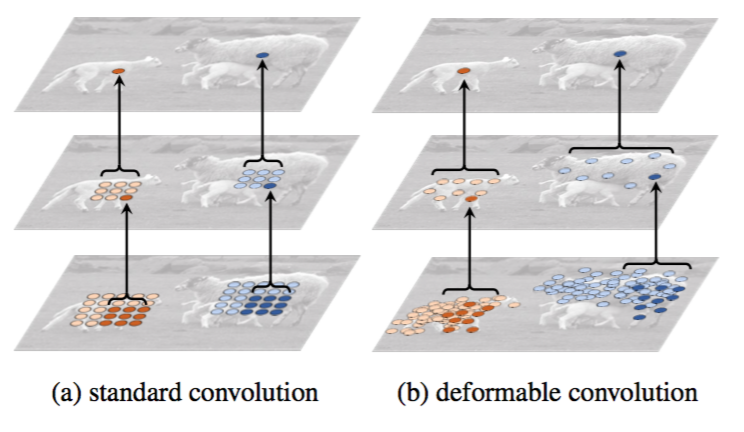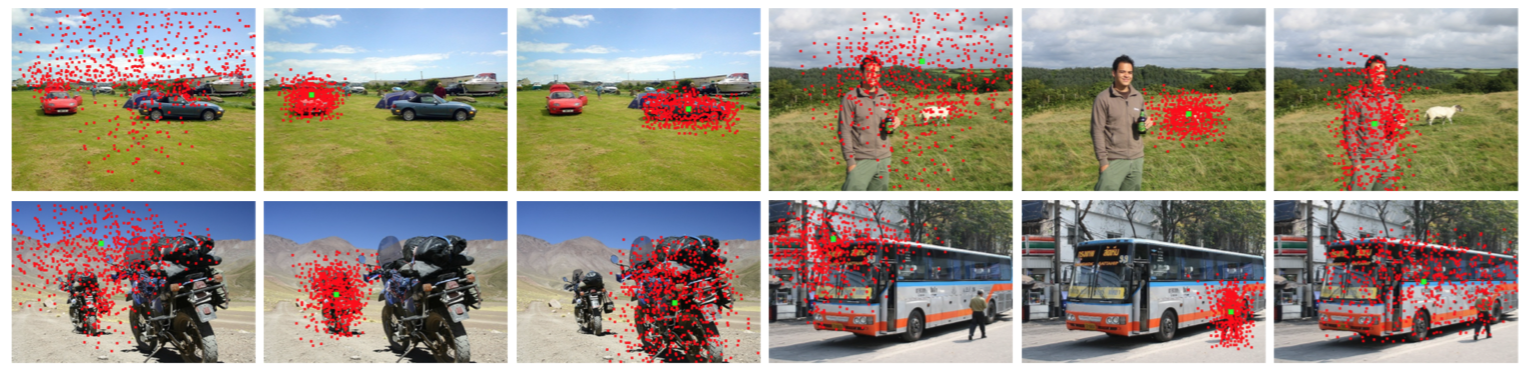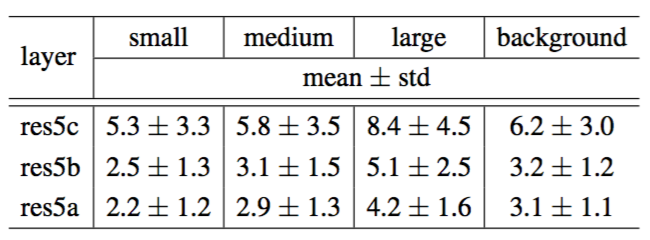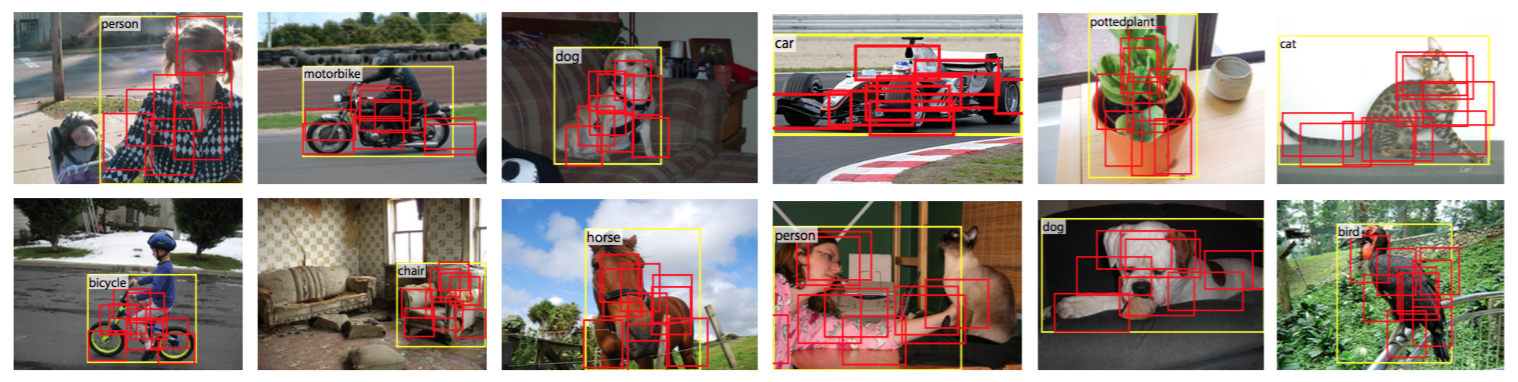3.1. 相关工作的背景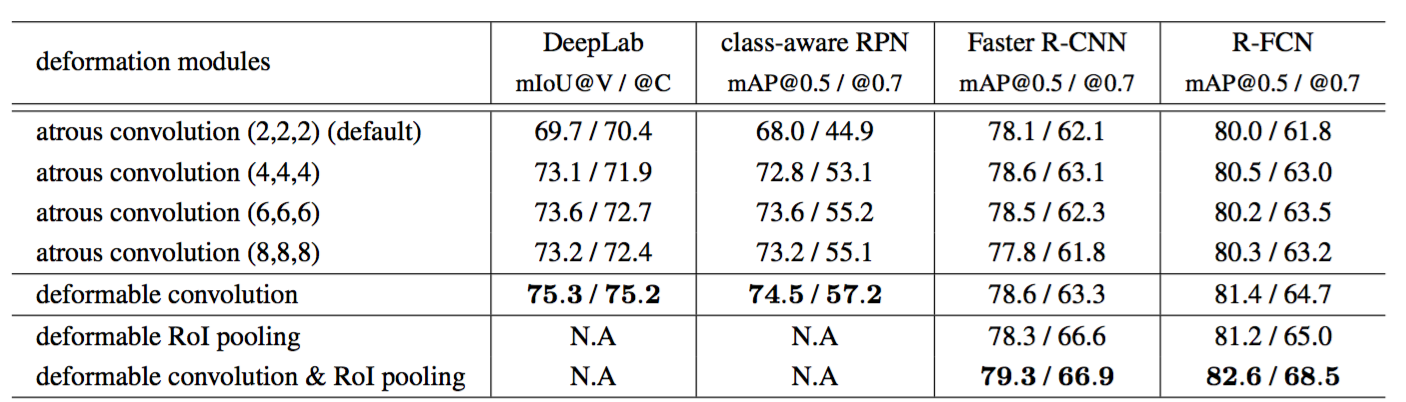DPM是一个浅层模型，其建模变形能力有限。虽然其推理算法可以通过将距离变换视为一个特殊的池化操作转换为CNN，但是它的训练不是端到端的，而是涉及启发式选择，例如选择组件和部件尺寸。相比之下，可变形ConvNets是深层的并进行端到端的训练。当多个可变形模块堆叠时，建模变形的能力变得更强。

DeepID-Net。它引入了一个变形约束池化层，它也考虑了目标检测的部分变形。因此，它与可变形RoI池化共享类似的精神，但是要复杂得多。这项工作是高度工程化并基于RCNN的。目前尚不清楚如何以端对端的方式将其应用于最近的最先进目标检测方法[47,7]。

RoI池化中的空间操作。空间金字塔池化在尺度上使用手工设计的池化区域。它是计算机视觉中的主要方法，也用于基于深度学习的目标检测[21,15]。

4. 实验

4.2. 消融研究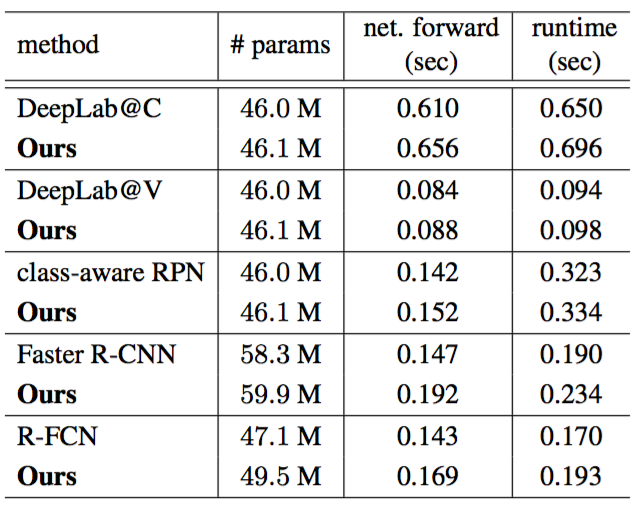4.3. COCO的目标检测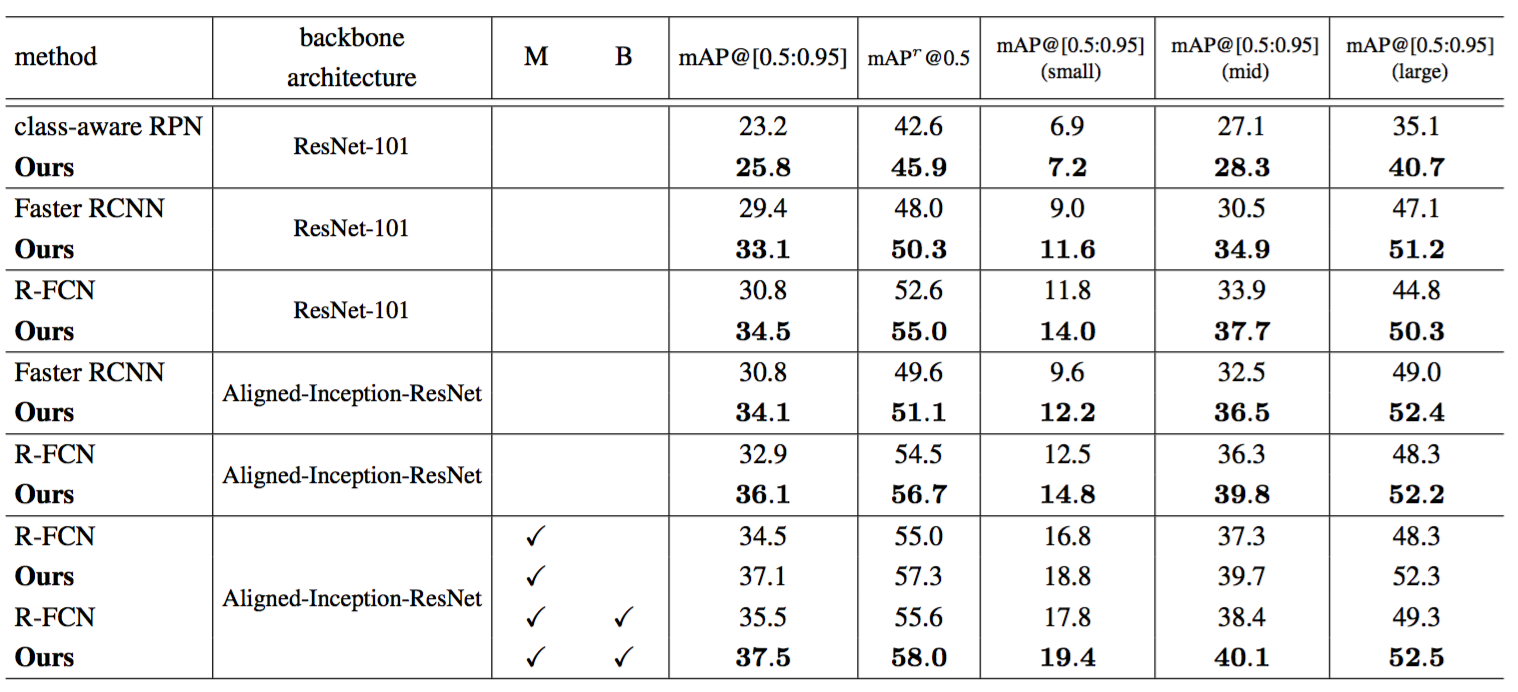致谢

Aligned-Inception-ResNet模型由Kaiming He，Xiangyu Zhang，Shaoqing Ren和Jian Sun在未发表的工作中进行了研究和训练。

 Y.-L. Boureau, J. Ponce, and Y. LeCun. A theoretical analysis of feature pooling in visual recognition. In ICML, 2010. 1

 B. D. Brabandere, X. Jia, T. Tuytelaars, and L. V. Gool. Dynamic filter networks. In NIPS, 2016. 6

 J. Bruna and S. Mallat. Invariant scattering convolution networks. TPAMI, 2013. 6

 L.-C. Chen, G. Papandreou, I. Kokkinos, K. Murphy, and A. L. Yuille. Semantic image segmentation with deep convolutional nets and fully connected crfs. In ICLR, 2015. 4, 7

 L.-C. Chen, G. Papandreou, I. Kokkinos, K. Murphy, and A. L. Yuille. Deeplab: Semantic image segmentation with deep convolutional nets, atrous convolution, and fully connected crfs. arXiv preprint arXiv:1606.00915, 2016. 4, 6, 7

 M. Cordts, M. Omran, S. Ramos, T. Rehfeld, M. Enzweiler, R. Benenson, U. Franke, S. Roth, and B. Schiele. The cityscapes dataset for semantic urban scene understanding. In CVPR, 2016. 7

 J. Dai, Y. Li, K. He, and J. Sun. R-fcn: Object detection via region-based fully convolutional networks. In NIPS, 2016. 1, 2, 3, 4, 5, 6

 J. Deng, W. Dong, R. Socher, L.-J. Li, K. Li, and L. Fei-Fei. Imagenet: A large-scale hierarchical image database. In CVPR, 2009. 4, 10

 S. Dieleman, J. D. Fauw, and K. Kavukcuoglu. Exploiting cyclic symmetry in convolutional neural networks. arXiv preprint arXiv:1602.02660, 2016. 6

 M. Everingham, L. Van Gool, C. K. Williams, J. Winn, and A. Zisserman. The PASCAL Visual Object Classes (VOC) Challenge. IJCV, 2010. 7

 P. F. Felzenszwalb, R. B. Girshick, D. McAllester, and D. Ramanan. Object detection with discriminatively trained part-based models. TPAMI, 2010. 2, 6

 W. T. Freeman and E. H. Adelson. The design and use of steerable filters. TPAMI, 1991. 6

 R. Gens and P. M. Domingos. Deep symmetry networks. In NIPS, 2014. 6

 S. Gidaris and N. Komodakis. Object detection via a multiregion & semantic segmentation-aware cnn model. In ICCV, 2015. 9

 R. Girshick. Fast R-CNN. In ICCV, 2015. 1, 2, 3, 6, 7

 R. Girshick, J. Donahue, T. Darrell, and J. Malik. Rich feature hierarchies for accurate object detection and semantic segmentation. In CVPR, 2014. 1, 3, 6

 R. Girshick, F. Iandola, T. Darrell, and J. Malik. Deformable part models are convolutional neural networks.

 K. He, X. Zhang, S. Ren, and J. Sun. Aligned-inceptionresnet model, unpublished work. 4, 10

 K. He, X. Zhang, S. Ren, and J. Sun. Spatial pyramid pooling in deep convolutional networks for visual recognition. In ECCV, 2014. 6

 K. He, X. Zhang, S. Ren, and J. Sun. Deep residual learning for image recognition. In CVPR, 2016. 4, 10

 M. Holschneider, R. Kronland-Martinet, J. Morlet, and P. Tchamitchian. A real-time algorithm for signal analysis with the help of the wavelet transform. Wavelets: Time-Frequency Methods and Phase Space, page 289297, 1989. 6

 J. Huang, V. Rathod, C. Sun, M. Zhu, A. Korattikara, A. Fathi, I. Fischer, Z. Wojna, Y. Song, S. Guadarrama, and K. Murphy. Speed/accuracy trade-offs for modern convolutional object detectors. arXiv preprint arXiv:1611.10012, 2016. 4

 J.-H. Jacobsen, J. van Gemert, Z. Lou, and A. W.M.Smeulders. Structured receptive fields in cnns. In CVPR, 2016. 6

 M. Jaderberg, K. Simonyan, A. Zisserman, and K. Kavukcuoglu. Spatial transformer networks. In NIPS, 2015. 2, 5

 Y. Jeon and J. Kim. Active convolution: Learning the shape of convolution for image classification. In CVPR, 2017. 5

 Y. Jia, C. Huang, and T. Darrell. Beyond spatial pyramids: Receptive field learning for pooled image features. In CVPR, 2012. 6

 A. Kanazawa, A. Sharma, and D. Jacobs. Locally scale-invariant convolutional neural networks. In NIPS, 2014. 6

 J. J. Koenderink and A. J. van Doom. Representation of local geometry in the visual system. Biological Cybernetics, 55(6):367–375, Mar. 1987. 6

 A. Krizhevsky, I. Sutskever, and G. E. Hinton. Imagenet classification with deep convolutional neural networks. In NIPS, 2012. 1

 D. Laptev and J. M. Buhmann. Transformation-invariantcon-volutional jungles. In CVPR, 2015. 6

 D. Laptev, N. Savinov, J. M. Buhmann, and M. Pollefeys. Ti-pooling: transformation-invariant pooling for feature learning in convolutional neural networks. arXiv preprint arXiv:1604.06318, 2016. 6

 S. Lazebnik, C. Schmid, and J. Ponce. Beyond bags of features: Spatial pyramid matching for recognizing natural scene categories. In CVPR, 2006. 6

 Y. LeCun and Y. Bengio. Convolutional networks for images, speech, and time series. The handbook of brain theory and neural networks, 1995. 1

 K. Lenc and A. Vedaldi. Understanding image representations by measuring their equivariance and equivalence. In CVPR, 2015. 6

 C.-H. Lin and S. Lucey. Inverse compositional spatial transformer networks. arXiv preprint arXiv:1612.03897, 2016. arXiv preprint arXiv:1409.5403, 2014. 6

 B. Hariharan, P. Arbeláez, L. Bourdev, S. Maji, and J. Malik. 5 Semantic contours from inverse detectors. In ICCV, 2011. 7  B. Hariharan, P. Arbeláez, R. Girshick, and J. Malik. Simultaneous detection and segmentation. In ECCV. 2014. 7

 T.-Y. Lin, P. Dollár, R. Girshick, K. He, B. Hariharan, and S. Belongie. Feature pyramid networks for object detection. In CVPR, 2017. 4, 7

 T.-Y. Lin, M. Maire, S. Belongie, J. Hays, P. Perona, D. Ramanan, P. Dollár, and C. L. Zitnick. Microsoft COCO: Common objects in context. In ECCV. 2014. 7

 W. Liu, D. Anguelov, D. Erhan, C. Szegedy, and S. Reed. Ssd: Single shot multibox detector. In ECCV, 2016. 1, 4

 J. Long, E. Shelhamer, and T. Darrell. Fully convolutional networks for semantic segmentation. In CVPR, 2015. 1, 6, 7

 D. G. Lowe. Object recognition from local scale-invariant features. In ICCV, 1999. 1, 6

 W. Luo, Y. Li, R. Urtasun, and R. Zemel. Understanding the effective receptive field in deep convolutional neural networks. arXiv preprint arXiv:1701.04128, 2017. 6

 W. Ouyang, X. Wang, X. Zeng, S. Qiu, P. Luo, Y. Tian, H. Li, S. Yang, Z. Wang, C.-C. Loy, and X. Tang. Deepid-net: Deformable deep convolutional neural networks for object detection. In CVPR, 2015. 6

 P. Perona. Deformable kernels for early vision. TPAMI, 1995. 6

 J. Redmon, S. Divvala, R. Girshick, and A. Farhadi. You only look once: Unified, real-time object detection. In CVPR, 2016. 1

 S. Ren, K. He, R. Girshick, and J. Sun. Faster R-CNN: Towards real-time object detection with region proposal networks. In NIPS, 2015. 1, 3, 4, 6, 7

 S. Ren, K. He, R. Girshick, and J. Sun. Faster R-CNN: Towards real-time object detection with region proposal networks. TPAMI, 2016. 7

 E. Rublee, V. Rabaud, K. Konolige, and G. Bradski. Orb: an efficient alternative to sift or surf. In ICCV, 2011. 6

 K. Sohn and H. Lee. Learning invariant representations with local transformations. In ICML, 2012. 6

 C. Szegedy, S. Ioffe, V. Vanhoucke, and A. Alemi. Inception-v4, inception-resnet and the impact of residual connections on learning. arXiv preprint arXiv:1602.07261, 2016. 4, 10

 C. Szegedy, S. Reed, D. Erhan, and D. Anguelov. Scalable, high-quality object detection. arXiv:1412.1441v2, 2014. 1

 D. E. Worrall, S. J. Garbin, D. Turmukhambetov, and G. J. Brostow. Harmonic networks: Deep translation and rotation equivariance. arXiv preprint arXiv:1612.04642, 2016. 6

 F. Yu and V. Koltun. Multi-scale context aggregation by dilated convolutions. In ICLR, 2016. 6

 F. Yu, V. Koltun, and T. Funkhouser. Dilated residual networks. In CVPR, 2017. 6